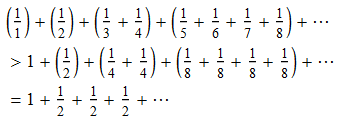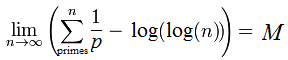# Generalising Erdős’ conjecture

It’s a well-known fact that the harmonic series (i.e. the sum of the reciprocals of the natural numbers) diverges to infinity. There are at least two reasonably straightforward ways to prove this, the first being the Cauchy condensation test. Essentially, in this case, it involves observing the following inequality:It can also be upper-bounded by a similar series, leading to the revelation that the series diverges logarithmically. Alternatively, you can obtain the result by comparing with the integral of 1/x. Specifically, the series can be expressed as the integral of a stepped function, trapped between the areas of two hyperbolae (which differ by 1).Hence, we can deduce that the following limit exists, and is bounded somewhere between 0 and 1:γ is the Euler-Mascheroni constant, which is roughly 0.577.

### Prime harmonic series

If we instead repeat this process with a subset of the natural numbers, the resulting series may converge. For instance, the sum of the reciprocals of the squares, known as ζ(2), converges to π²/6. What about the sum of the reciprocals of the primes? This turns out to diverge, albeit extremely slowly. Euler provided a heuristic argument (it lacks the rigour to be an actual proof) that it diverges at an asymptotic rate of log(log(n)). With a little work, it’s possible to rigorously prove that the sums of reciprocals of primes is bounded below by log(log(n)) − 1:Indeed, there’s an analogy of the Euler-Mascheroni constant called the Meissel-Mertens constant:The previous proof demonstrates that M ≥ −1. In fact, our lower bound is quite poor, as M is approximately equal to 0.2615.

### Brun’s constant

The sum of the reciprocals of Mersenne primes is obviously convergent, since it can be bounded above by the series 1 + 1/2 + 1/4 + 1/8 + …, which converges to 1. It transpires that the sum of the reciprocals of twin primes also converges (the proof is much more difficult); the limit of this series is Brun’s constant. When computing the twin primes, Professor Thomas Nicely discovered a bug in the floating-point arithmetic of the Pentium processor.

### Green-Tao theorem and Erdős’ conjecture

The τ theorem (named after Professors Ben Green and Terry Tao) states that there exist arbitrarily long arithmetic progressions in the prime numbers. For example, a sequence of 26 primes in arithmetic progression is {43142746595714191, 48425980631694091, 53709214667673991, 58992448703653891, 64275682739633791, 69558916775613691, 74842150811593591, 80125384847573491, 85408618883553391, 90691852919533291, 95975086955513191, 101258320991493091, 106541555027472991, 111824789063452891, 117108023099432791, 122391257135412691, 127674491171392591, 132957725207372491, 138240959243352391, 143524193279332291, 148807427315312191, 154090661351292091, 159373895387271991, 164657129423251891, 169940363459231791, 175223597495211691} (sequence A204189 in the OEIS). On the other hand, there are no infinite arithmetic progressions of primes; the proof of this is trivial.

The primes are described as a substantial set, which means that the sum of their reciprocals diverges. Erdős conjectured that the Green-Tao theorem generalises to all substantial sets, offering a prize of 3000 USD for a proof. This conjecture would generalise Szemerédi’s theorem (since all sets of positive lower density are substantial), and therefore van der Waerden’s theorem (since in any k-colouring of the integers, we can find a colour with a lower density at least 1/k). For an explanation of these theorems, together with proofs of some other generalisations, consult the third chapter (Combinatorics II) of MODA.

### Generalising further?

In the same way that the Hales-Jewett theorem generalises van der Waerden’s theorem, and that the density analogue of Hales-Jewett extends Szemerédi’s theorem, it seems natural to consider this (conjectural) generalisation of Erdős’ conjecture:

• Suppose we have a set A of natural numbers, such that the sum of the reciprocals of elements of A diverges. Write the elements of A in base n, for n ≥ 2. Then I conjecture that there exists a combinatorial line of length n.

For example, it conjectures the existence of an arithmetic progression of 10 primes, which form a combinatorial line when written in decimal. Problem 51 of Project Euler asks you to find the first partial combinatorial line of 8 primes, and provides an explicit example (56**3) with 7 primes: {56003, 56113, 56333, 56443, 56663, 56773, 56993}. If you can find a combinatorial line of 10 primes, I’ll be impressed.

One way to avoid all combinatorial lines is to take the integers whose decimal expansions do not contain any 9s. This sequence begins {1,2,3,4,5,6,7,8,10,11,12,…}. If the sum of the reciprocals of these integers diverged, then we would have a simple counter-example to my conjecture. However, it is straightforward to prove that the sum of the reciprocals of 9-less integers (the Kempner series) converges; the limit is roughly 22.92. The proof generalises in the obvious way to any combination of radix and digit.

This entry was posted in Uncategorized. Bookmark the permalink.

### 0 Responses to Generalising Erdős’ conjecture

1.apgoucher says:

Update: Keith F. Lynch has now discovered a combinatorial line of 10 primes, namely 39402*9*7*3, where the asterisk successively takes the values {0, 1, 2, 3, 4, 5, 6, 7, 8, 9}.

2.http://essaysmonster.net/ says:

Thanks for helpful paper. I like log)))

3.http://iessays-writer.org says:

Thanks so much for this wonderful website as well as this post. This post is the kind of thing That keeps me on track through.

4.essaycapital.org says:

cool

5.Chris Evans Jacket says:

Thank for all your efforts, keep posting this kind of articles!

•EMF says:

SPAM SPAM SPAM SPAM